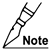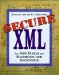# 6.5 Function Library

All XPath implementations must include certain core functions. For particular applications, this function library may be augmented. For example:

• XML canonicalization adds the function "here( )". (See Chapter 9.)

• XPointer adds several functions and data types. (See Chapter 7.)

Of course, the various operators that can appear in XPath expressions may also be considered as functions invoked by the operator's syntax rather than the usual functional syntax. XPath does not consider the node tests listed in Section 6.3.3, which look like function calls (such as "text( )"), to actually be functions.

The rest of this section describes the functions in function prototype format. An italicized data type before the function name gives the type of output. The function name appears in boldface type. Each parameter is represented by its italicized data type. Where a question mark ("?") appears after a parameter data type, it indicates that the parameter is optional.

#### 6.5.1 Node-Set Functions

Node-set-related functions are listed here in alphabetic order by function name, followed by a description of their output.The "id" function, in its simplest mode with a string parameter, and the "count" function are particularly important to XML Security.

number count (node-set)

The number of nodes in the parameter.

node-set id (object)

The element with a unique ID equal to the parameter if the parameter is a string. If the parameter is a node-set, it consists of the set of elements, each with a unique ID equal to the string value of any member of the node-set. For other parameter types:

• The parameter is converted to its string value, which is treated as a list of tokens separated by white space

• The result is the set of elements with unique IDs equal to any of those tokens

number last( )

A number equal to the context size of the expression evaluation context.

string local-name(node-set?)

The local part of the expanded name of the first node, in document order, in the parameter node-set. If the parameter node-set is null or if the first node has no expanded name, the function returns a null string. If the parameter is omitted, the function acts as if the parameter were a node-set containing only the context node. For example:

` local-name ( <foo:bar xmlns:foo="http://xyz.example"/> ) = "bar" `

string name (node-set?)

The prefix-qualified name representing the first node, in document order, in the parameter node-set. If the parameter node-set is null or if the first node has no expanded name, the function returns a null string. If the parameter is omitted, the function acts as if the parameter were a node-set containing only the context node. For example:

` name ( <foo:bar xmlns:foo="http://xyz.example"/> ) = "foo:bar" `In the case where multiple prefixes are bound by namespace declarations to the same URI, XPath permits the return of any of these prefixes as part of the qualified name. However, XML Security requires that the prefix returned always be the original prefix appearing in the XML before conversion to an XPath node-set. Otherwise, conversion of XML to an XPath node-set would destroy the information regarding what prefix was originally used. As a consequence, the XPath node-set could not be printed consistently.

string namespace-uri (node-set?)

The URI of the expanded name of the first node, in document order, in the parameter node-set. (It will be null except for element and attribute nodes that have namespaces.) If the parameter node-set is null or if the first node has no expanded name, the function returns a null string. If the parameter is omitted, the function acts as if the parameter were a node-set containing only the context node. For example:

` namespace-uri ( <foo:bar xmlns:foo="http://xyz.example"/> )               = "http://xyz.example" `

number position( )

A number equal to the context position of the expression evaluation context.

#### 6.5.2 String Functions

The string functions either return a string result or require string parameters. They are listed in alphabetic order here by function name, followed by a description of their output.

string concat (string, string, string*)

The concatenation of its parameters. The "*" indicates that additional string parameters may be present.

boolean contains (string, string)

True if its second parameter appears as a substring of its first parameter; otherwise, false.

string normalize-space (string?)

Its parameter, or the string value of the context node if its parameter is omitted, with leading and trailing white space deleted and each internal contiguous run of white space converted to one space.

boolean starts-with (string, string)

True if its first parameter starts with its second parameter; otherwise, false.

string string (object?)

Converts the object to a string. If the object is a node-set, the function returns the string value of the first node in the set in document order or the empty string if the node-set is empty.

number string-length (string?)

The length of the parameter or, if the parameter is omitted, the length of the string value of the context node.

string substring (string, number, number?)

That part of its first parameter starting at the position specified by the second parameter and continuing for the third parameter number of characters or until the end of the first parameter if the function call omits the third parameter. Following are some examples:

` substring ("12345", 2, 2) = "23" substring ("12345", 4, 99) = "45" substring ("12345", -1, 3) = "1" `

string substring-after (string, string)

The remainder of the first parameter after the second parameter's first occurrence within the first parameter, or the empty string if there are no such occurrences.

string substring-before (string, string)

The part of the first parameter that comes before the second parameter's first occurrence within the first parameter, or the empty string if there are no such occurrences.

string translate (string, string, string)

Returns the first parameter with occurrences of characters that also appear in the second parameter replaced by the corresponding character in the third parameter. If no corresponding character occurs in the third parameter, because the third parameter is shorter than the second parameter, the character is simply deleted from the first parameter. For example:

` translate ( "123456", "156", "IV") = "I234V" `

#### 6.5.3 Boolean Functions

Boolean functions yield a Boolean result. They are listed alphabetically by function name here, followed by a description of their return value.

boolean boolean (object)

Converts its parameter to a Boolean value according to the following rules:

• A node-set is true only if it is nonempty.

• The Boolean function passes through its parameter if that parameter is a Boolean value.

• A string is true only if it is of nonzero length.

• A number is true only if it is nonzero and not NaN (Not a Number) [IEEE 754].

• For other types of parameters, the results depend on the type.

boolean false( )

Returns the "false" Boolean value.

boolean lang (string)

The parameter must be a language tag [RFC 1766]. This function returns true if the context node's language is the same as or is a more specific language than the parameter. The language of the context node is determined by looking for an xml:lang attribute there or at the nearest ancestor node with an xml:lang attribute. If no xml:lang attribute can be found, the function returns the "false" Boolean value.

Language tags consist of a primary tag followed by zero or more subtags separated by hyphens. All tags are case insensitive for example, "fr," "NO-nynorsk," and "en-US-brooklyn." A language tag is a more specific language tag than the parameter if it consists of the parameter extended by one or more subtags.

boolean not (boolean)

Returns true if its parameter is false; otherwise, returns false.

boolean true( )

Returns the "true" Boolean value.

#### 6.5.4 Number Functions

Number functions yield a numeric result. They are listed alphabetically by function name here, followed by a description of their return value.

number ceiling (number)

Returns the smallest (closest to minus infinity) integer that is not less than its parameter.

number floor (number)

Returns the largest (closest to plus infinity) integer that is not greater than its parameter.

number number (object?)

Converts its parameter to a number and then returns that number. If the optional parameter is omitted, the function acts as if that parameter were the node-set consisting of the context node. Conversion of various types of parameters occurs as follows:

• Boolean "true" and "false" values are converted to 1 and 0, respectively.

• A string representing a number is converted to the nearest [IEEE 754] number. Leading and trailing white space characters are ignored. Other strings are converted to NaN (Not a Number).

• A node-set is first converted to a string, as if by a call to the "string" function, then that string is converted as above.

• All other types of parameters are converted in a type-dependent fashion.

number round (number)

Returns the integer that is closest to its parameter. If the parameter is exactly midway between two integers, it is rounded toward plus infinity.

number sum (node-set)

Converts each node in the parameter into its string value, converts that value to a number, and finally returns their sum.Secure XML: The New Syntax for Signatures and Encryption
ISBN: 0201756056
EAN: 2147483647
Year: 2005
Pages: 186